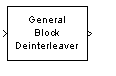# General Block Deinterleaver

Restore ordering of symbols in input vector

## Library

Block sublibrary of Interleaving

•## Description

The General Block Deinterleaver block rearranges the elements of its input vector without repeating or omitting any elements. If the input contains N elements, then the Permutation vector parameter is a column vector of length N. The column vector indicates the indices, in order, of the output elements that came from the input vector. That is, for each integer k between 1 and N,

Output(Permutation vector(k)) = Input(k)

The Permutation vector parameter must contain unique integers between 1 and N.

Both the input and the Permutation vector parameter must be column vector signals.

This block can output sequences that vary in length during simulation. For more information about sequences that vary in length, or variable-size signals, see Variable-Size Signal Basics (Simulink).

This block accept the following data types: `int8`, `uint8`, `int16`, `uint16`, `int32`, `uint32`, `boolean`, `single`, `double`, and fixed-point. The output signal inherits its data type from the input signal.

To use this block as an inverse of the General Block Interleaver block, use the same Permutation vector parameter in both blocks. In that case, the two blocks are inverses in the sense that applying the General Block Interleaver block followed by the General Block Deinterleaver block leaves data unchanged.

## Parameters

Permutation vector source

A selection that specifies the source of the permutation vector. The source can be either `Dialog` or ```Input port```. The default value is `Dialog`.

Permutation vector

A vector of length N that lists the indices of the output elements that came from the input vector. This parameter is available only when Permutation vector source is set to `Dialog`.

## Examples

This example reverses the operation in the example on the General Block Interleaver block reference page. If you set Permutation vector to `[4,1,3,2]'` and you set the General Block Deinterleaver block input to `[1;40;59;32]`, then the output of the General Block Deinterleaver block is `[40;32;59;1]`.

## Pair Block

General Block Interleaver

## Extended Capabilities

### Functions

Introduced before R2006a